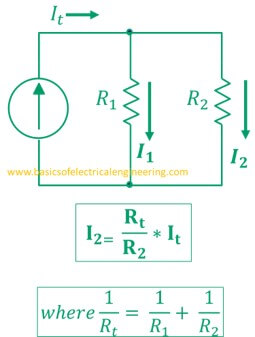# Basics of Electrical Engineering

Learn the basics of Electrical Engineering.

In parallel electrical circuits, the current doesn't remain same. The current divide rule is used to find the divided current in parallel circuits. What you'll learn:
1. The statement of current divider
2. General formula
3. Formula for two resistors with Examples
4. Formula for three resistors with Examples
5. Its derivation

### Statement and formula of Current Divider

Statement: The electrical current entering the node of a parallel circuit is divided into the branches. Current divider formula is employed to calculate the magnitude of divided current in the circuits.
Let's understand the basic definitions:
Node: A point where two or more than two components are joined.
Parallel circuit: The circuit in which one end of all components share a common node, and the other end of all components share the other common node. You can learn more about parallel circuit configuration here.

### General formula

A parallel circuit with 'n' number of resistors and an input voltage source is illustrated below. We are interested to find the current which is flowing through Rx.In the above formula:
Ix: The current through Rx.
It: The total current which enters the circuit.
Rx: The resistance of the component whose current value is to be determined
Rt: The equivalent resistance of the parallel circuit

#### For two resistors

Let's consider a parallel circuit having two resistors R1 and R2. The current It enters the node. We are interested to calculate the current that is flowing through. The general formula and circuit now take the form:We can modify the previous equation to obtain an alternative formula:

Let's solve an example to better understand the formulas.

Example # 1: A 5 kΩ resistor connects in parallel to a 20 kΩ resistor. 5 A current enters the node. Find the current across both resistors.
Solution:#### Current divider rule for three resistors

Let's consider the third case where we have three parallel resistors. The easy method which should be followed here is to find the equivalent resistance first, and then to apply the original formula:

#### Derivation of Current Divider formula

The derivation of CDR formula is very simple. Let's reconsider the general circuit:
Apply the Ohm's law on Rx.
Ix = E/R
where E = ItRt.
Ix = ItRt/Rx.
or
Ix = [Rt/Rx] * It
So that was all about the Current Divider.
You can practice some more examples here to develop more concepts and knowledge on Current Divider circuits:

#### 14 comments:

1.Very good. Thanks for this post. Do more like this.

2.3.How this Rt became 80ohms ? From those R1 R2 R3

1.1/Rt= 1/R1= 1/R2= 1/R3
THEN TAKE RESIPROCAL TO CHANGE 1/RT INTO RT

2.SOORY 1/R1+1/R2+1/R3 AND THEN TAKE RECIPROCAL

4.Hi, thanks a lot for this information. I loves to read this blog. Keep it up
By the way, visit the new post at my blog Best books for iit jee preparation to get free ebooks in reference of academic engineering field and highly competitive engineering exams.

5.Very Nice Illustration.

6.thanks, this was helpful

7.A battery is connected to a resistance causing a current of 0.5A in the circuit. The current
drops to 0.4 when an additional resistance of 5? is connected in series . The current will drop to
0.2A when the resistance is further increased by

1.8.ITS GOOD I THINK BETTER

9.Nice Post Illustration

10.Hey, this day is too much good for me, since this time I am reading this enormous informative article here at my home. Thanks a lot for massive hard work. ground fault current transformer

11.https://electric-shocks.com/voltage-and-current-divider-rule-vdr-cdr-series-parallel-circuit/